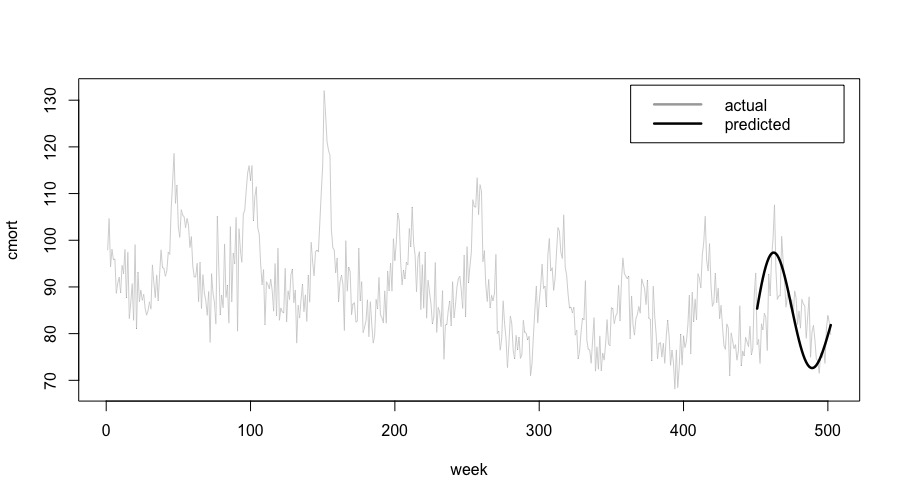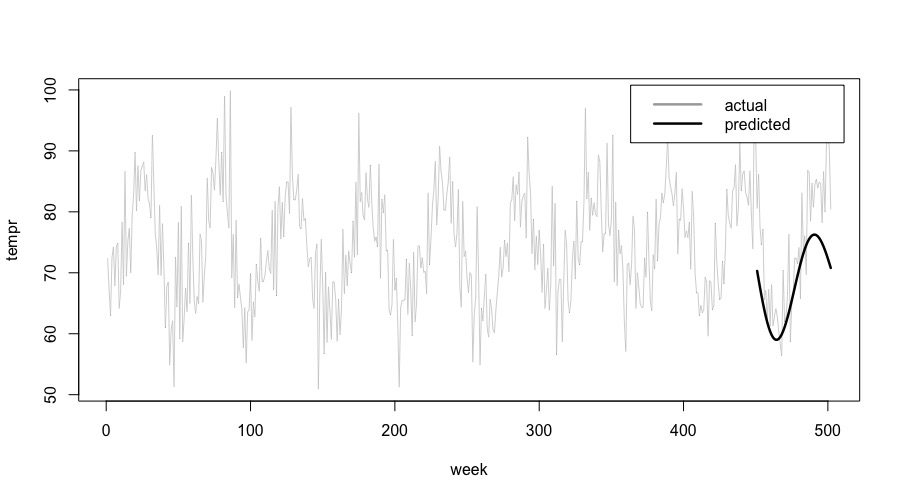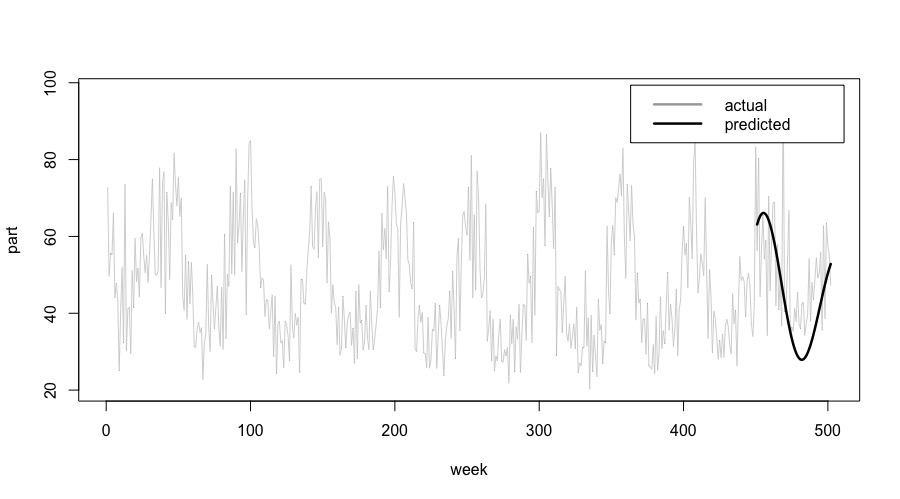# Use VARMAX with Teradata R Package - 16.20 - Using VARMAX with Teradata R Package - Teradata R Package

## Teradata® R Package User Guide

Product
Release Number
16.20
Published
February 2020
Language
English (United States)
Last Update
2022-05-02
dita:mapPath
qbt1519078127352.ditamap
dita:ditavalPath
Generic_no_ie_no_tempfilter.ditaval

This example uses the dataset "lap", found in the R package "astsa". The dataset consists of 508 mean weekly observations of eleven variables including three measurements of human death rates (total mortality, cardiacrelated mortality, and respiratory-related mortality) and eight atmospheric measurements (temperature, relative humidity, carbon monoxide, sulphur dioxide, nitrogen dioxide, hydrocarbons, ozone, and particulates).

The VARMAX function is used to predict three of the time series in the dataset: cardiac mortality ('cmort'), temperature ('tempr') and particulates ('part').

In this example, you first run the VARMAX function on a shortened version of the dataset - the first 456 points, and then plot the predicted values versus the actual values for the final fifty-two weeks for each of the three times series.

1. Install the "astsa" package on your R client, if it's not already installed. And load the library.
`install.packages('astsa')`
`library(astsa)`
2. Load the data set, and parametrize the different set sizes.
```lap_df <- as.data.frame(lap)

nData <- nrow(lap_df)
nShortData <- nData - 52```
3. Add "seqid" and "id" columns to prepare the data frame for use with the VARMAX function.
```seqid <- rep(1, nData)
id <- seq(1:nData)

lap_df_indexed <- cbind(seqid, id, lap_df)```
4. Create a table in the database to hold the data.
`copy_to(con, lap_df_indexed, name="lap_data", overwrite=TRUE)`
5. Create a tibble for the main data set.
`tddf_lap_data <- tbl(con, "lap_data")`
6. Obtain the shortened data by using the first nShortData points of the main dataset. Then create a tibble for the shortened dataset.
```df_lap_data <- as.data.frame(tddf_lap_data)
df_lap_data_short <- df_lap_data[1:nShortData,]

copy_to(con, df_lap_data_short, name="lap_data_short", overwrite=TRUE)

tddf_lap_data_short <- tbl(con, "lap_data_short")```
7. Perform the VARMAX analysis with the td_varmax_mle() analytic function.
```lap_varmax <- td_varmax_mle(
data = tddf_lap_data_short,
response.columns = c("cmort","tempr","part"),
partition.columns = c("id"),
orders = "1, 0, 1",
data.partition.column = c("seqid"),
data.order.column = c("id")
)```
8. The results include the VARMAX coefficients, goodness of fit measurements, and whether the function converged, in addition to the fifty-two predicted values for the three response columns. Save the results as a table in the database and convert the table to an R data frame.
```copy_to(con, lap_varmax\$result, name="lap_results", overwrite=TRUE)

tddf_lap_results = tbl(con, "lap_results")

results_df <- as.data.frame(tddf_lap_results)```
In the output data frame "results_df", the 'stepahead' column is the week number. To create the plots in the following steps, rows need to be ordered by the week number.
As the 'stepahead' column is of character type, it must be transformed to numeric for proper ordering.
9. Transform the 'stepahead' column into numeric type.
`results_df <- transform(results_df, stepahead = as.numeric(stepahead))`
10. Order the "results_df" rows by the 'stepahead' week column.
`results_df <- results_df[order(results_df\$stepahead),]`
After the transformation, predicted values in "results_df" are in rows 1 to 52.
11. Retrieve the step ahead predictions.
`cmort_pred <- results_df[1:52, 'predict_cmort']`
`tempr_pred <- results_df[1:52, 'predict_tempr']`
`part_pred <- results_df[1:52, 'predict_part']`
12. Create a data frame consisting of the first 450 values of 'cmort', 'tempr' and 'part' with the actual and predicted values for the final fifty-two values.
```df_short <- lap[1:450, c("cmort","tempr","part")]
df_na <- rep(NA, 450)
df_pred <- cbind(cmort_pred, tempr_pred, part_pred)
df_actual <- lap[451:502,c("cmort","tempr","part")]

weeks_1to450 <- cbind(df_short, df_na, df_na, df_na)
weeks_451to502 <- cbind(df_actual, df_pred)

df_complete <- rbind(weeks_1to450, weeks_451to502)```
13. Convert the data frame into time series class to use the time series plotting functions.
`ts_complete <- ts(df_complete)`
14. Plot the results.
1. Plot the cardiac mortality data 'cmort' to compare the predicted values to the actual values.
```ts.plot(ts_complete[,c(1,4)],
gpars=list(col=c("darkgray","black"), lwd=c(0.5,2.5), ylab="cmort", xlab="week"))

legend("topright", lty = 1,
lwd=2.5,col=c("darkgray","black"),legend=c("actual","predicted"), inset = .02)```2. Plot the temperature 'tempr' data to compare the predicted values to the actual values.
```ts.plot(ts_complete[,c(2,5)],
gpars=list(col=c("darkgray","black"), lwd=c(0.5,2.5), ylab="tempr", xlab="week"))

legend("topright", lty = 1,
lwd=2.5,col=c("darkgray","black"),legend=c("actual","predicted"), inset = .02)```3. Plot the particulates 'part' data to compare the predicted values to the actual values.
```ts.plot(ts_complete[,c(3,6)],
gpars=list(col=c("darkgray","black"), lwd=c(0.5,2.5), ylab="part", xlab="week"))

legend("topright", lty = 1,
lwd=2.5,col=c("darkgray","black"),legend=c("actual","predicted"), inset = .02)```